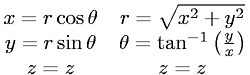Equations > Geometry > Coordinate Systems > Cartesian to Cylindrical Coordinates (3-D)

Cartesian to Cylindrical Coordinates (3-D)Latex Code:

MathML Code:

 $\begin{array}{ccc}\hfill x=r\mathrm{cos}\theta \hfill & \hfill r=\sqrt{{x}^{2}+{y}^{2}}\hfill & \hfill \hfill \\ \hfill y=r\mathrm{sin}\theta \hfill & \hfill \theta ={\mathrm{tan}}^{-1}\left(\fracyx\right)\hfill & \hfill \hfill \\ \hfill z=z\hfill & \hfill z=z\hfill & \hfill \hfill \end{array}$

MathType 5.0: